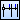# Creating Ordinate Linear Dimension

Create an ordinate linear dimension by locating dimension lines continuously on the same line in the horizontal/vertical direction using the start point as the base point.
Depending on the position of the end point against the start point, the direction of the ordinate linear dimension (horizontal/vertical) is determined. When creating a dimension line horizontally or vertically fixed, create a baseline linear dimension with Ordinate Horizontal Dimension or Ordinate Vertical Dimension command.

1. Point [Ordinate Dimension] on [Shape] menu, and click [Ordinate Linear Dimension].
Then you will be able to create an ordinate linear dimension.
2.  Supplemental ▪ You can enter into the ordinate linear dimension creating status by clickingon [Shape] toolbar.
3. Click the start point of an ordinate linear dimension on the drawing.
4.  Supplemental ▪ You can also specify the start and end points of the first dimension, followed by the end points of the second and subsequent dimensions by switching [Start Point] box to [Base Point Array] box.
5. Click the end point of the first dimension line on the drawing.
The length between the start and end points will be automatically set as a dimension value.
6. Specify how to rotate the entire dimension shape in [Rotate] box on Command Window.
Automatic
Automatically determines whether it is horizontal or vertical.
Horizontal
The dimensions will be horizontal.
Vertical
The dimensions will be vertical.
Fit to Shape
Fits to the same angle as the selected dimension Shape.
Manual
Specifies the angle of the extension line arbitrarily.
7. Specify an input method for dimension values in [Input Dimension Value] box on Command Window.
Auto
Dimension values will be automatically input.
Manual
Dimension values will be manually input. Input a dimension value in [Dimension Value Character] box.
8. Specify whether to locate the dimension value on the auxiliary line on the end point side, or on the end point side of the dimension line, in [Dimension Value Place] box on Command Window.
9. Click a position where a dimension line is to be located on the drawing.
A dimension line will be located at the clicked position.
10. Click end points of the second and subsequent dimension lines on the drawing in order.
Each time you click the end point, dimension lines using the start point as the base will be created continuously on the same line, for example the dimension line connecting the secondly-specified end point and start point, that connecting the third-specified end point and start point, and so on.
The second and subsequent dimension lines will be created in the direction you specify the end point of the first dimension line (horizontally/vertically).
11. Press Enter key on the keyboard, or clickon the toolbar on Command Window.
Specifying end points will be complete, and an ordinate linear dimension will be created.
 Supplemental ▪ You can also specify the distance from the start/end point to the first dimension line by switching [Locate Point] box to [Distance from Base Point] box. In such a case, specify a point locating the dimension line in [Direction Point] box. ▪ You can specify the start point, end point, locate point, direction point and end point of after second dimension by inputting X and Y coordinates in [Start Point], [End Point], [Locate Point], [Direction Point] and [End Point for Second and Subsequent Dimensions] boxes on Command Window.